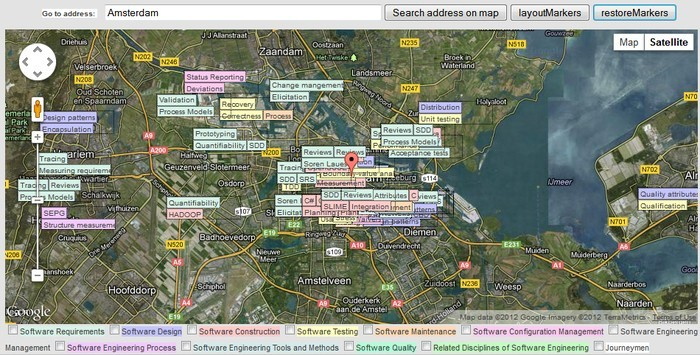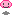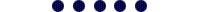Neither one nor Many

September 23 2012

I have an unfinished project with--in general--some really horrible sourcecode, but with some cool functions and solutions I came up with as well. One thing I needed for example was to calculate latitude and longitude coordinates from X and Y positions on a google maps canvas, taking zoom level into account. I could not find these conversion functions (around august 2011 anyway).

[Edit, now since May 21, 2015 Google Maps Api V3 was released, which makes it possible with the Google API. I also found an example gist here]

## Aligning markers to a grid

The reason I needed these convertion functions in the first place was for creating an align feature for (custom) markers on a google maps canvas. This is how it works before/after aligning:The (very simple) algorithm I came up with divides the map in slots. A grid with a width of 100 for example, only positions markers on 100, 200, 300, 400 pixels. In this example, the 'nearest' slot's width of a marker at position 220,50 pixels wouuld be 200.

The algorithm in pseudocode:

``````INITIALIZE GRID HEIGHT AND WIDTH ACCORDING TO MAP'S ZOOMLEVEL
/* gridwidth = 100 << (21 - map.getZoom()) */
/* gridheight = 30 << (21 - map.getZoom()) */

FOREACH MARKER

CONVERT MARKER LAT,LON TO X,Y COORDINATES
/* y = latToY(marker.getPosition().lat()); */
/* x = lonToX(marker.getPosition().lng()); */

CONVERT X,Y TO NEAREST SLOT X,Y
/* slot_y = Math.round(y - (y % gridheight)) */
/* slot_x = Math.round(x - (x % gridwidth)) */

SET MARKER X,Y TO SLOT X,Y

WHILE SLOT POSITION IS OCCUPIED BY ANOTHER MARKER

MOVE TO NEXT SLOT POSITION
/* Next slot position is according to a simple spiral movement  */

SET MARKER X,Y TO SLOT X,Y

ENDWHILE

CONVERT MARKER X,Y TO LAT,LON

STORE SOMEWHERE THAT MARKER IS IN THIS SLOT POSITION

ENDFOREACH``````

: The search for next slot position is according to this pattern:

up, right, down, down, left, left, up, up, up, right, right, right, etc.

• The change of direction is continious (a spiral): {up, right, down, left, ..}
• The number of 'steps' in each direction is {1, 1, 2, 2, .... n, n}

## latToX() and lonToY()

I'm not an expert in math but I was able to find some expressions online that resolved lat+lon for x+y (the other way around). I simply replaced all the constants with their values and put them in a solver to solve them for the variables I was interested in (e.g. longitude for XtoLon). I probably have the sites bookmarked somewhere but I can't find them.

``````var glOffset = 268435456;
var glRadius = 85445659.4471;// offset / pi

function lonToX(lon)
{
var p = Math.PI / 180;
var b = glRadius * lon;
var c = b * p;
return Math.round(glOffset + c);
}

function XtoLon(x)
{
return -180 + 0.0000006705522537 * x;
}

function latToY(lat)
{
Math.log((1 + Math.sin(lat * Math.PI / 180)) /
(1 - Math.sin(lat * Math.PI / 180))) / 2);
}

function YtoLat(y)
{
var e = 2.7182818284590452353602875;
var a = 268435456;
var b = 85445659.4471;
var c = 0.017453292519943;

return Math.asin(Math.pow(e,(2*a/b-2*y/b))/(Math.pow(e,(2*a/b-2*y/b))+1)-1/(Math.pow(e,(2*a/b-2*y/b))+1))/c;
}
``````

They are not pretty but I like them because they work really well## Edit 10-AUG-2015: deltaLonPerDeltaX(), deltaLatPerDeltaY()

I found out somebody on Stackoverflow elaborated my functions with a deltaLonPerDeltaX() and deltaLatPerDeltaY(). The original poster's image is no longer available, so I'm not sure if I understand the question correctly, and therefore these additional functions. But there is a nice extra info cited from Google, which I will copy here:

``At zoom level 1, the map consists of 4 256x256 pixels tiles, resulting in a pixel space from 512x512. At zoom level 19, each x and y pixel on the map can be referenced using a value between 0 and 256 * 2^19``Comment may not be visible immediately, because I process everything manually.**

**) I plan to automate this.., but it's on my ToDo since for ever..

Author:Ray Burgemeestre
february 23th, 1984

Topics:
C++, Linux, Webdev

Other interests:
Music, Art, Zen